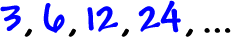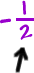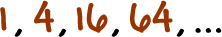If you're going on to Calculus, these are going to be important!

Remember that with arithmetic sequences we added something each time.

With geometric sequences, we'll multiply by something each time.

Here are some geometric sequences:We multiplied by 2 each time.We multiplied byeach time. This is called the ratio.

So, how do we find the ratio?

Sometimes, you can just look...The ratio is 4.

But, you usually don't get this lucky.